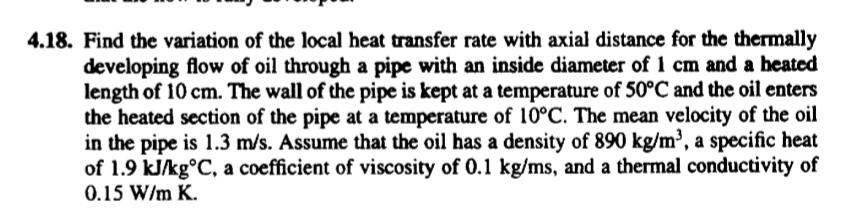# Question Solved1 Answer4.18. Find the variation of the local heat transfer rate with axial distance for the thermally developing flow of oil through a pipe with an inside diameter of 1 cm and a beated length of 10 cm. The wall of the pipe is kept at a temperature of 50°C and the oil enters the heated section of the pipe at a temperature of 10°C. The mean velocity of the oil in the pipe is 1.3 m/s. Assume that the oil has a density of 890 kg/m”, a specific heat of 1.9 kJ/kg°C, a coefficient of viscosity of 0.1 kg/ms, and a thermal conductivity of 0.15 W/m K.P0Q4CQ The Asker · Mechanical EngineeringTranscribed Image Text: 4.18. Find the variation of the local heat transfer rate with axial distance for the thermally developing flow of oil through a pipe with an inside diameter of 1 cm and a beated length of 10 cm. The wall of the pipe is kept at a temperature of 50°C and the oil enters the heated section of the pipe at a temperature of 10°C. The mean velocity of the oil in the pipe is 1.3 m/s. Assume that the oil has a density of 890 kg/m”, a specific heat of 1.9 kJ/kg°C, a coefficient of viscosity of 0.1 kg/ms, and a thermal conductivity of 0.15 W/m K.
More
Transcribed Image Text: 4.18. Find the variation of the local heat transfer rate with axial distance for the thermally developing flow of oil through a pipe with an inside diameter of 1 cm and a beated length of 10 cm. The wall of the pipe is kept at a temperature of 50°C and the oil enters the heated section of the pipe at a temperature of 10°C. The mean velocity of the oil in the pipe is 1.3 m/s. Assume that the oil has a density of 890 kg/m”, a specific heat of 1.9 kJ/kg°C, a coefficient of viscosity of 0.1 kg/ms, and a thermal conductivity of 0.15 W/m K.